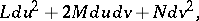# Bonnet theorem

(diff) ← Older revision | Latest revision (diff) | Newer revision → (diff)

Bonnet's theorem on the existence and the uniqueness of a surface with given first and second fundamental forms . Let the following two quadratic forms be given:the first one of which is positive definite, and let the coefficients of these forms satisfy the Gauss equations (cf. Gauss theorem) and the Peterson–Codazzi equations. Then there exists a surface, which is unique up to motions in space, for which these forms are, respectively, the first and the second fundamental forms.

Bonnet's theorem on the diameter of an oval surface: If the curvature of an oval surface is larger than or equal toat all its points, then the external diameter of this surface is smaller than; this estimate cannot be improved. Stated by O. Bonnet in 1855.